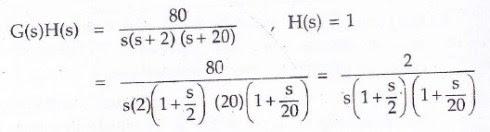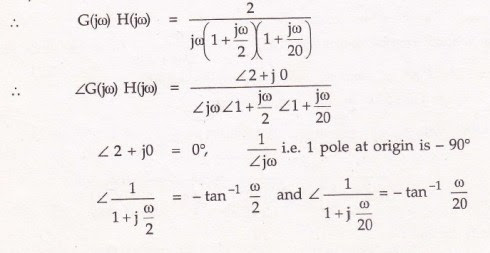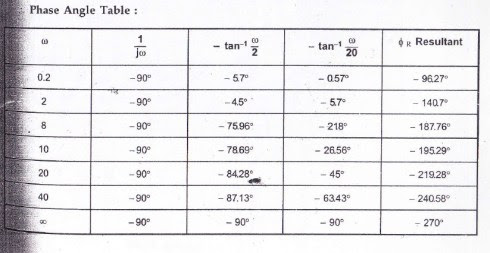How to draw bode plot in control system

How to draw a Bode plot on semi-log graph paper

Question: A unity control feedback system has G(s) =80/s(s+2)(s+20). Draw the bode plot. Determine the gain margin and phase margin. Also determine gain cross-over frequency and phase cross-over frequency. Comment on the system stability using this Bode plot.

Solution:

Step 1:

Arrange G(s)H(s)in time constant form as follows:Step 2:

Identify factors of given H(s)G(s):

1)      K=2

2)      One pole is at origin. (Because there is ‘s’ in the denominator).

3)      Simple pole 1/(1+s/2) with T1 = ½. Hence wc1 = 1/T1 = 2.

4)      Similarly simple pole 1/(1+s/20) with T2 = 1/20. wc2 = 1/T2 = 20.

Step 3: Magnitude plot analysis:

1)      For K=2, draw a line of 20logK = 20log2 = 6 dB.

2)      For one pole at origin. Straight line of slope -20 dB/decade passing through intersection point of w=1 and 0 dB. ( Trick to remember: if there is no pole at origin draw a straight line. For simple pole draw -20dB/decade line, for second order pole i.e. ‘s^2 term in denominator’ draw -40dB/decade line, for third order pole (i.e. S^3)draw -60dB/decade line and so on. And always take intersection point of w=1 and 0 dB. )

3)      Shift intersection point of w=1 and 0 dB on 20logK line and draw parallel to -20 dB/decade line drawn. This will continue as a resultant of K and 1/s till first corner frequency occurs. i.e. wc2=2.

4)      At wc1 = 2, as there is simple pole it will contribute the rate of -20 dB/decade hence resultant slope after wc1 = 2 becomes -20-20=-40 dB/decade. This is addition of K, 1/s and 1/(1+s/2). This will continue till it intersects next corner frequency line i.e. wc2=20.

5)      At wc2 = 20, there is simple pole contributing -20dB/ decade and hence resultant slope after wc2=20 becomes -40-20=-60 dB/decade. This is resultant of overall G(s)H(s). i.e. G(jw)H(jw) the final slope is -60dB/decade, as there is no other factor present.

Step 4: Phase angle plot: Convert G(s)H(s) to G(jw)H(jw)

To draw straight line of -40 dB/decade and -60 dB/decade from wc1 = 2 and wc2 = 20, draw faint lines of slope -20, -40, -60 dB/decade from intersection point of w=1 and 0 dB line and just draw parallel to them from respective points .Step 5: Bode plot and solution:

You may also like:

1.Thank you!

2.3.Can u Solove without zero example

4.Mohammed HanneefMay 4, 2018 at 4:25 PM

this is of great help for a quick refresh.
thank you

5.This problem is from which book ?